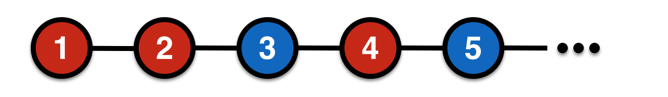# Coloring With Pythagorean TripletsCan the set of all positive integers be colored such that each integer is colored either red or blue and all Pythagorean triples include at least one integer of each color?

For example, if 3 and 5 were blue, 4 would have to be red since $3^2+4^2 = 5^2.$

Note: This problem is not reasonably solvable by hand, but the answer is now known. For a giveaway, see this paper.

×